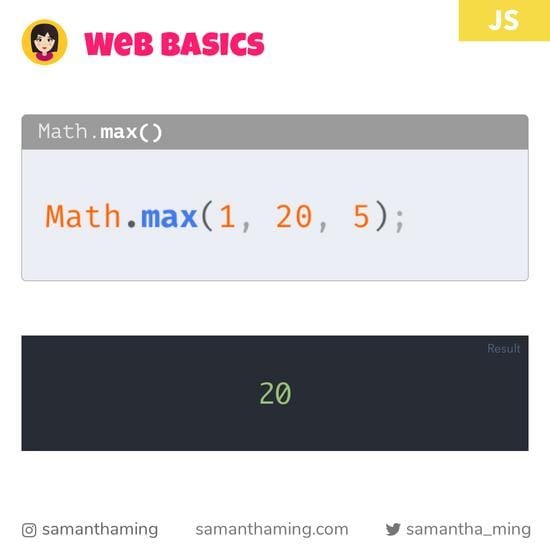🏗 Site is currently under construction 🏗

# # Math.max()

In today's lesson, let's learn about `Math.max()`. This is part of my Web Bascis series, where I cover essential programming topics every web developer should absolutely know!

Ever wonder how we can get largest number from a set of numbers. Look no futher, that's what this function is about!

``````Math.max(1, 20, 5);

// 20
``````

## # Examples

Yup, it also works with negative numbers.

``````Math.max(-1, -20, -5);

// -1
``````

### # Math.max with Arrays

Now, passing individual numbers can be a bit tedious. More helpful is to use this to find the largest number of an array. To do that, we can use ES6 Spread.

``````const array = [1, 20, 5];
const max = Math.max(...array);

max; // 20
``````

## # Resources